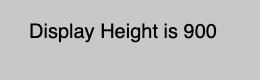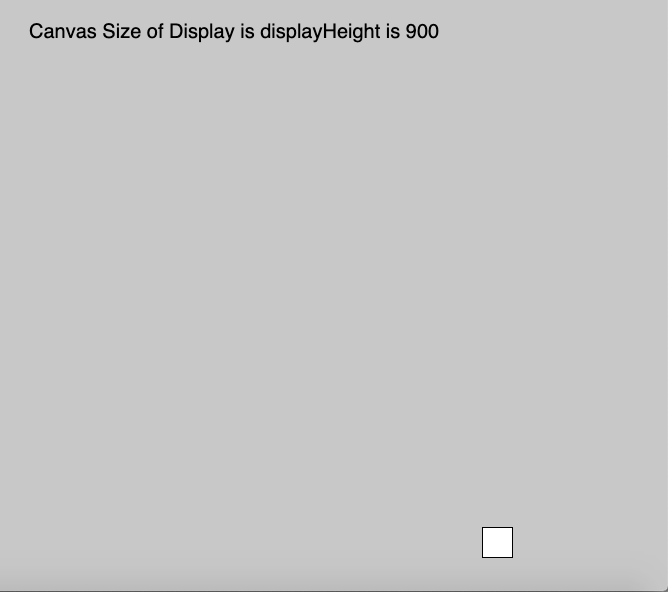Open In App
Related Articles
• Write an Interview Experience
• p5.js
• p5.js Introduction

# p5.js displayHeight Variable

The displayHeight variable in p5.js is used to store the height of the screen display of the device. The value of height is stored according to the default pixelDensity. This variable is used to run a full-screen program on any display size. Multiply it with pixelDensity to return the actual screen size.

Syntax:

```displayHeight
```

Parameters: This function does not accept any parameter.

Below program illustrates the displayHeight variable in p5.js:
Example-1:

 `function` `setup() {``   ` `    ``createCanvas(1000, 400);``   ` `    ``// Set text size to 40px``    ``textSize(20); ``}`` ` `function` `draw() {``    ``background(200);``    ``rect(mouseX, mouseY, 30, 30);`` ` `    ``//Use of displayHeight Variable``    ``text(``"Display Height is "` `+ displayHeight, 30, 40);``}`

Output:Example-2:

 `function` `setup() {`` ` `    ``createCanvas(1000, displayHeight);`` ` `    ``// Set text size to 40px``    ``textSize(20);``}`` ` `function` `draw() {`` ` `    ``background(200);``    ``rect(mouseX, mouseY, 30, 30);`` ` `    ``//Use of displayHeight Variable``    ``text(``"Canvas Size of Display is displayHeight is "` `         ``+ displayHeight, 30, 40);``}`

Output: